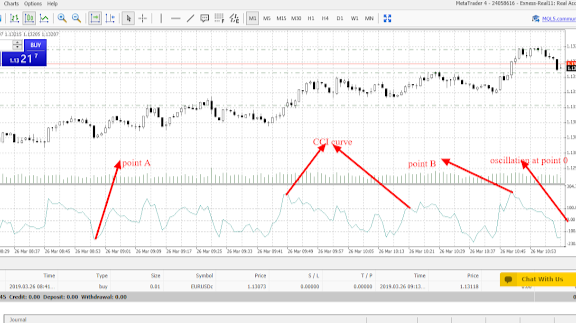# Commodity Channel Index(CCI): How to analyze the market using Commodity Channel Index

Commodity channel index was developed by Donald Lambert with the main objective of measuring the deviation of a commodity price from its average statistical price. According to Donald Lambert, he suggested that when the value of the commodity channel index is high, that is an indication that the price is also high while when the value of the commodity channel index is low that is an indication that the price is also low. Donald lambert further derived the commodity channel index formulae as follows;

• Finding the typical price by adding the high, the low and the close price of each bar and then divide the whole result by 3.This is as follows;

TP=(HIGH+LOW+CLOSE)/3

• calculating the n-period SIMPLE MOVING AVERAGE of the typical price. This is as follows;

SMA(TP,N)=SUM(TP,N)/N

• Subtraction SMA(TP, N) from typical prices of each of preceding n-periods.This is as follows;

D= TP-SMA(TP,N)

• Calculating the n-period SIMPLE MOVING AVERAGE of absolute D values. This is as follows;

SMA(D,N)=SUM(D,N)/N

• Multiplying the received SMA(D, N) by 0.015. This is as follows;

M=SMA(D,N)*0.015

• commodity channel index(CCI) is then gotten by dividing M by D.This is as follows;

CCI= M/D

Whereas;

HIGH is maximum bar price

LOW is minimum bar price

CLOSE is a close price

SMA is Simple Moving Average

N is number of periods used for calculation while;

SUM is the sum.

Since commodity channel index is an oscillator it, therefore, has an oscillation at point 0 where the CCI oscillate above or below. When the CCI curve is above +100(positive 100 ) that is an indication that there is an overbought in the market while when the CCI curve is below -100(negative 100) that is an indication that there is an oversold in the market. At overbought market which is above 100,the CCI curve will signal the trader to close any buy position and open a sell position since the market will start moving downwards while at oversold market which is below -100, the CCI curve will signal the trader to close any sell position and open a buy position since the market will start moving upwards. This is indicated as from the candlestick chart below;

From the candlestick chart above, the commodity channel index curve is indicated by the two red joining arrows. The oscillation point 0 is indicated by the first red arrow. There are two points, point A and point B. At point A the CCI is below -100 thus an indication of the oversold market thus signaling the trader to close any sell position and enter a buy position since the market has started moving upwards as indicated. At point B the CCI is above 100 thus an indication of the overbought market thus signaling the trader to close any buy position and enter a sell position since the market has started moving downwards.

### Recommendation: if you are a day trader just use 1 min,5 min,15 min and 30 min time frame while if you are a swing trader just use 1 hour and above time frame if you want commodity channel index indicator to work well for you.

If you would like to support our work, here is our tipping details;

skrill donation[email protected]

Neteller donation[email protected]

Bitcoin :1Fp5aLgRB6WJnC7nxGw57M3JbbexaAWHG2
Ethereum; 0x346570c491b76c7cd51699bdd272762111b743a9
Litecoin: LeEH7B82ccXJ6QoibCryGJNpDGNx2x77fL
Dogecoin: DLShs2vqhMc1ggqo8MPPMBTcPAZdqCZoWdTrader, Blockchain Technologist and Contentpreneur. Also founder and CEO @ Quinto Trader